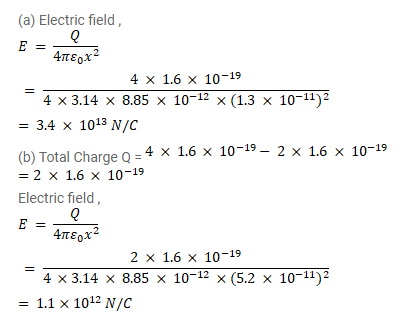# Consider the following very rough model of a beryllium atom.Question:

Consider the following very rough model of a beryllium atom. The nucleus has four protons and four neutrons confined to a small volume of radius $10^{-15} \mathrm{~m}$. The two $1 \mathrm{~s}$ electrons make a spherical charge cloud at an average distance of $1.3 \times 10^{-11} \mathrm{~m}$ from the nucleus, whereas the two 2 s electrons make another spherical cloud at an average distance of $5.2 \times 10^{-11} \mathrm{~m}$ from the nucleus. Find the electric field at (a) a point just inside the $1 \mathrm{~s}$ cloud and (b) a point just inside the $2 \mathrm{~s}$ cloud.

Solution: